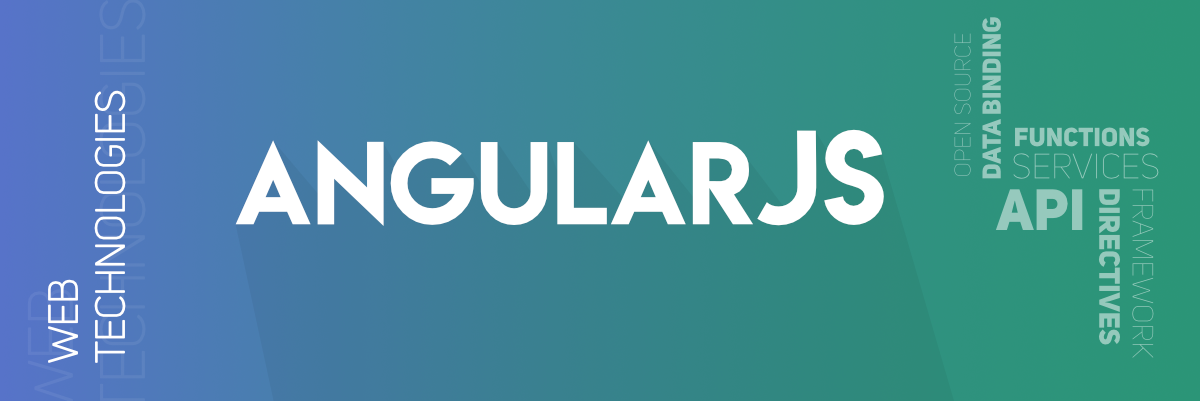# What are the AngularJs Global API ?

Global API in AngularJS: API stands for Application Programming Interface. It is a set of protocols, routines, and tools for building software applications that allow the user to interact with the application and perform several tasks. In AngularJS Global API is a set of global Javascript functions used for performing tasks like comparing objects, iterating objects, and converting data.Some API functions in AngularJS are shown below:

angular.lowercase: This is a built-in filter that is used to transform any string into lowercase.

Syntax:

`angular.lowercase(string);`

Example 1: This example describes the basic usage of the Global API that is utilized to convert the string to lowercase.

## HTML

 ` ` `<``html``> ` ` `  `<``head``> ` `    ``<``script` `src``= ` `"https://ajax.googleapis.com/ajax/libs/angularjs/1.6.9/angular.min.js"``> ` `    `` ` ` ` ` `  `<``body``> ` `    ``<``div` `ng-app``=``"App"` `ng-controller``=``"Ctrl"``> ` `        ``<``p``>{{"Before Conversion: " + i1 }} ` `        ``<``p``>{{"After Conversion: " + i2 }} ` `    `` ` `    ``<``script``> ` `        ``var app = angular.module('App', []); ` `        ``app.controller('Ctrl', function(\$scope) { ` `            ``\$scope.i1 = "GeeksforGeeks"; ` `            ``// converting string into lowercase ` `            ``\$scope.i2 = angular.lowercase(\$scope.i1); ` `        ``}); ` `    `` ` ` ` ``

Output:

```Before Conversion: GeeksforGeeks
After Conversion: geeksforgeeks```

angular.uppercase: This is a built-in filter that is used to transform any string into uppercase.

Syntax:

`angular.uppercase(string);`

Example 2: This example describes the basic usage of the Global API that is utilized to convert the string to uppercase.

## HTML

 ` ` `<``html``> ` ` `  `<``head``> ` `    ``<``script` `src``= ` `"https://ajax.googleapis.com/ajax/libs/angularjs/1.6.9/angular.min.js"``> ` `    `` ` ` ` ` `  `<``body``> ` `    ``<``div` `ng-app``=``"App"` `ng-controller``=``"Ctrl"``> ` `        ``<``p``>{{"Before Conversion: " + i1 }} ` `        ``<``p``>{{"After Conversion: " + i2 }} ` `    `` ` `    ``<``script``> ` `        ``var app = angular.module('App', []); ` `        ``app.controller('Ctrl', function(\$scope) { ` `            ``\$scope.i1 = "geeksforGeeks"; ` `             `  `            ``// converting string into uppercase ` `            ``\$scope.i2 = angular.uppercase(\$scope.i1); ` `        ``}); ` `    `` ` ` ` ` `  ``

Output:

```Before Conversion: geeksforgeeks
After Conversion: GEEKSFORGEEKS```

angular.isString: This is a built-in filter that is used to check whether the given value is a string or not, if the value is a string then it returns true else it returns false

Syntax:

`angular.isString(value);`

Example 3: This example describes the use of the Global API that is utilized to check whether the entered value is a string or not.

## HTML

 ` ` `<``html``> ` ` `  `<``head``> ` `    ``<``script` `src``= ` `"https://ajax.googleapis.com/ajax/libs/angularjs/1.6.9/angular.min.js"``> ` `    `` ` ` ` ` `  `<``body``> ` `    ``<``div` `ng-app``=``"App"` `ng-controller``=``"Ctrl"``> ` `        ``<``p``>{{"Value is: " + i1 }} ` `        ``<``p``>{{"Value is string: " + i2 }} ` `    `` ` `    ``<``script``> ` `        ``var app = angular.module('App', []); ` `        ``app.controller('Ctrl', function(\$scope) { ` `            ``\$scope.i1 = 15; ` `           `  `            ``// checks whether the given value is a string ` `            ``\$scope.i2 = angular.isString(\$scope.i1); ` `        ``}); ` `    `` ` ` ` ``

Output:

```Value is: 15
Value is String: false```

angular.isNumber: This is a built-in filter that is used to check whether the given value is a number or not, if it is a number then it returns true else it returns false.

Syntax:

`angular.isNumber(value);`

Example 4: This example describes the use of the Global API that is utilized to check whether the given value is a number or not.

## HTML

 ` ` `<``html``> ` ` `  `<``head``> ` `    ``<``script` `src``= ` `"https://ajax.googleapis.com/ajax/libs/angularjs/1.6.9/angular.min.js"``> ` `    `` ` ` ` ` `  `<``body``> ` `    ``<``div` `ng-app``=``"App"` `ng-controller``=``"Ctrl"``> ` `        ``<``p``>{{"Value is: " + i1 }} ` `        ``<``p``>{{"Value is string: " + i2 }} ` `    `` ` `    ``<``script``> ` `        ``var app = angular.module('App', []); ` `        ``app.controller('Ctrl', function(\$scope) { ` `            ``\$scope.i1 = 15; ` `           `  `            ``// checks whether the given value is a number ` `            ``\$scope.i2 = angular.isNumber(\$scope.i1); ` `        ``}); ` `    `` ` ` ` ``

Output:

```Value is: 15
Value is Number: true```

There are several other Global APIs, which are described below:

Whether you're preparing for your first job interview or aiming to upskill in this ever-evolving tech landscape, GeeksforGeeks Courses are your key to success. We provide top-quality content at affordable prices, all geared towards accelerating your growth in a time-bound manner. Join the millions we've already empowered, and we're here to do the same for you. Don't miss out - check it out now!

Previous
Next